# Java IO Character Streams Tutorial with Examples

#### View more Tutorials: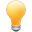Follow us on our fanpages to receive notifications every time there are new articles.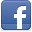Facebook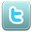Twitter

## 1- Introduction

In the previous instruction document, I introduced input-output binary stream. You need to understand it before studying input-output character stream. You can see here:

## 2- The difference between binary streams and character streams

Binary Stream, each one reads/writes a byte (equivalent to 8 bits)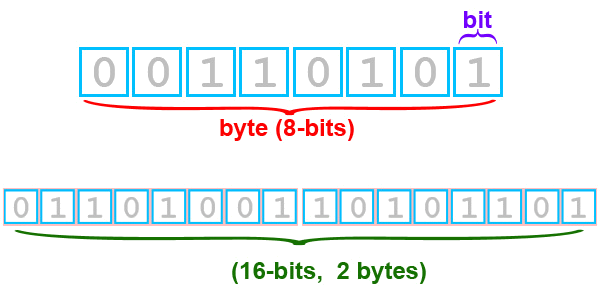Meanwhile, character stream read/write a character. It is dependent on type of encoding (UTF-8, UTF-16,...) to decide the number of bytes in each reading/writing turn which are 1 byte, 2 bytes, or 3 bytes. Let's see the following illustrated image:
UTF-16:
This is a Japanese text. If it is stored in a File encoded UTF-16, bytes in hard disk drive will be similar to the illustrated image:
• Two first bytes (254,255) mean notifying the beginning of a String with UTF-16 encoding.
• The next characters are encoded by 2 bytes.
• For example, 'J' character is encoded by 2 bytes (0 and 74)
• 'P' character is encoded by 2 bytes (0 and 80)
• .....
• When reading from file with UTF-16 encoding, it will ignore two first bytes and read two consecutive bytes into one character.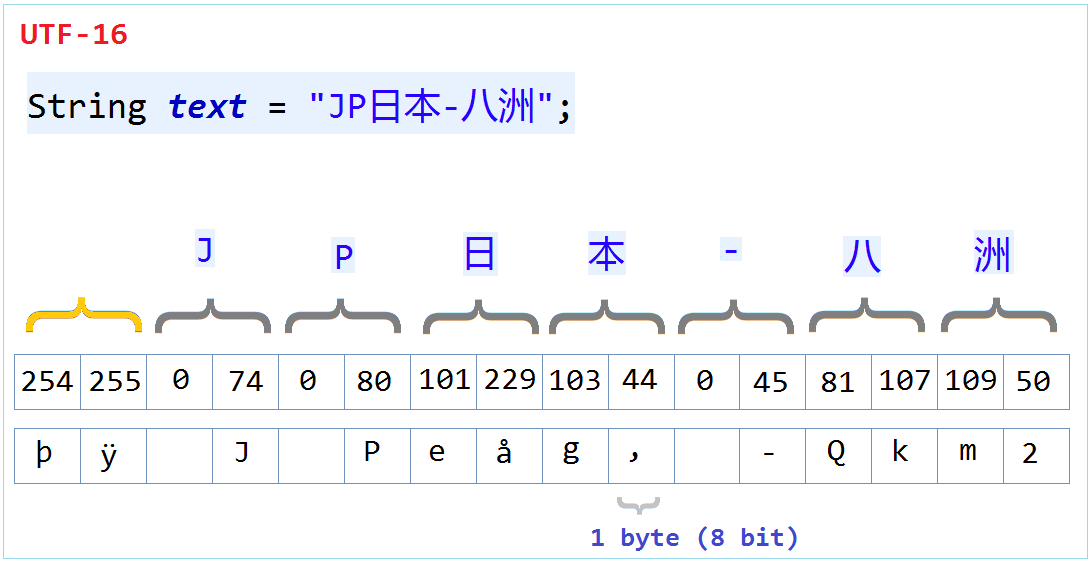UTF-8:
It will be different if the same above-mentioned Japanese text is encoded by UTF-8. You can see that bytes are stored in hard disk drive:
• ​With ASCII characters, it will only use one byte for storage.
• For example, it takes 1 byte to store 'J' character (74)
• It takes 1 byte to store 'P' character (80)
• It may take 2 bytes or 3 bytes to store other characters.
• In the rule of reading, base on a UTF-8 Table.
• Read the first byte, if it <= 127, then it's an ASCII character.
• Else if it >127, then it needs to read the second byte, and check whether two bytes can be combined into one character based on the UTF-8 Table.
• If the first 2 bytes do not correspond to 1 character, It will read the next byte and combine them into a character.
• UTF-8 uses up to 3 bytes to store a character.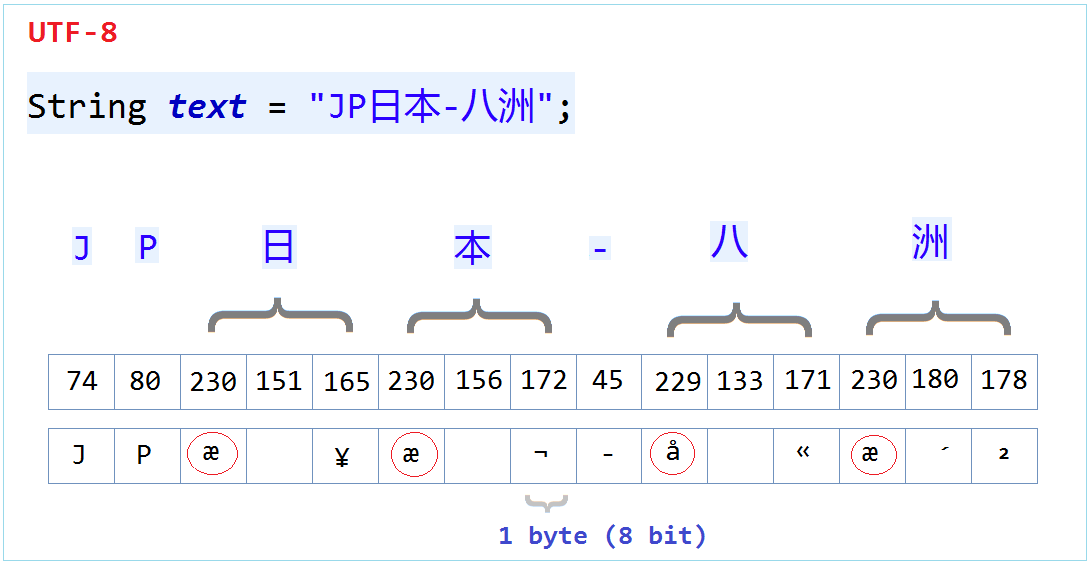To sum up, when you save a document with any encoding, you need to read with an equivalent encoding, or else the output of reading will be wrong.

## 3- Overview of the character streams

Hierarchies of Classes: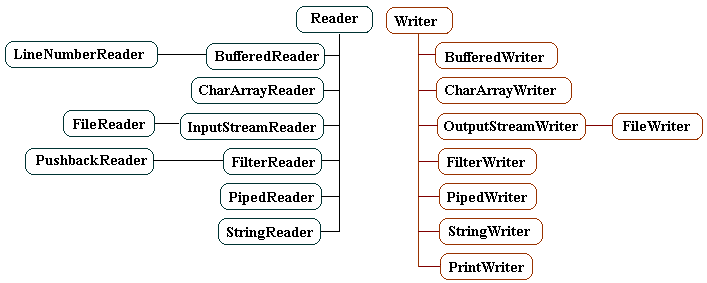Reader is a abstract class. it is the base class for character reading streams.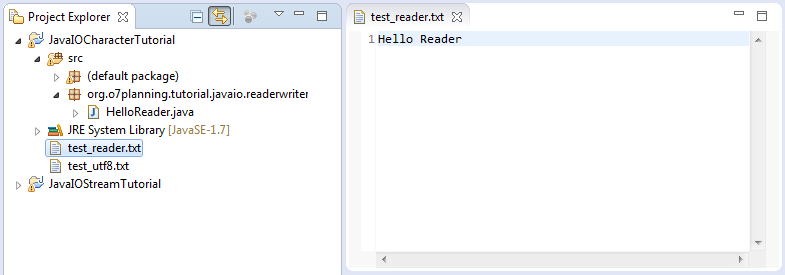``````

import java.io.IOException;

public static void main(String[] args) throws IOException {
// With default encoding.
int i = -1;

// Read each character in turn.
while ((i = r.read()) != -1) {
// Cast to char.
System.out.println((char) i);
}
r.close();
}

}
``````
Run the example: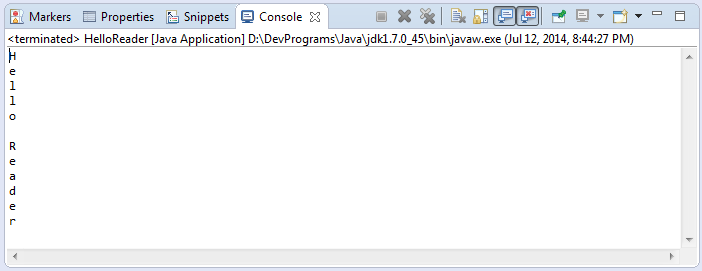The next example is to read many characters in a reading turn. This helps enhance the efficiency of the program compared to reading each character in turn.
``````

import java.io.IOException;

// This example, read multi characters in once.

public static void main(String[] args) throws IOException {

// With default encoding.
// Create temporary array of characters.
char[] temp = new char;
int i = -1;

// Read multiple characters at once,
// and assign them to the elements of the array.
// Return the number of characters read.
// Or returns -1 if the end of the stream has been reached.
while ((i = r.read(temp)) != -1) {
String s = new String(temp, 0, i);
System.out.println(s);
}
r.close();
}

}
``````

## 5- Class java.io.Writer

Writer is an abstract class, it is the base class for the character writing stream.
HelloWriter.java
``````

import java.io.File;
import java.io.FileWriter;
import java.io.IOException;
import java.io.Writer;

public class HelloWriter {

public static void main(String[] args) throws IOException {
File dir = new File("C:/test");

// Create 'C:/test' directory, if it does not exists.
dir.mkdirs();

// Create a Writer, to write data to the file.
// Using default encoding.
Writer w = new FileWriter("C:/test/test_writer.txt");

// Array of characters.
char[] chars = new char[] { 'H', 'e', 'l', 'l', 'o', //
' ', 'w', 'r', 'i', 't', 'e', 'r' };

// Write characters to stream.
for (int i = 0; i < chars.length; i++) {
char ch = chars[i];
int j = (int) ch;
//
w.write(j);
}
// Close stream,
w.close();
}
}
``````
Results of running the example: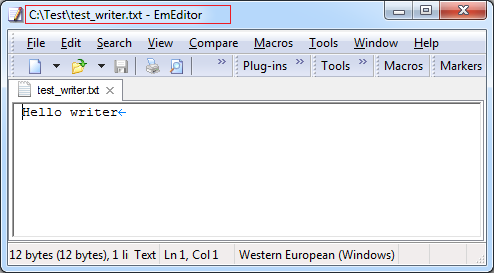The next example is to write many characters in a stream at the same time. Specifically, we write an array of characters into a stream. This helps enhance the efficiency of the program compared to writing each character in turn.
HelloWriter2.java
``````

import java.io.File;
import java.io.FileWriter;
import java.io.IOException;
import java.io.Writer;

public class HelloWriter2 {

public static void main(String[] args) throws IOException {

File dir = new File("C:/test");
// Create 'C:/test' directory, if it does not exists.
dir.mkdirs();

// Create a Writer, to write the data to the file.
Writer w = new FileWriter("C:/test/test_writer2.txt");

//
char[] chars = new char[] { 'H', 'e', 'l', 'l', 'o', //
' ', 'w', 'r', 'i', 't', 'e', 'r' };

// Write all characters to the stream.
w.write(chars);

// Java often uses buffers to store data temporarily.
// When the buffer is full, it flush the data to the file.
// You can proactively flush data into the file.
w.flush();

// Write 'new line' character to stream.
w.write('\n');

String s = "FileWriter";

// Write a String to stream.
w.write(s);

// Close stream.
// It will push the data in buffer to the file.
// Also finish writing data.
w.close();
}
}
``````
Results of running the example: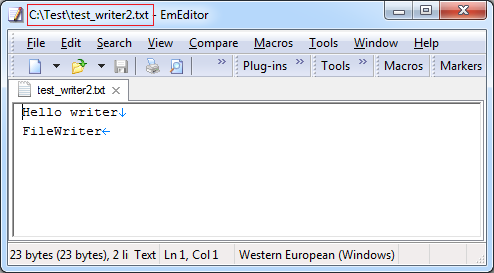## 6- How a binary stream is converted into a character stream?

You have a binary stream. And you want to convert it into a character stream?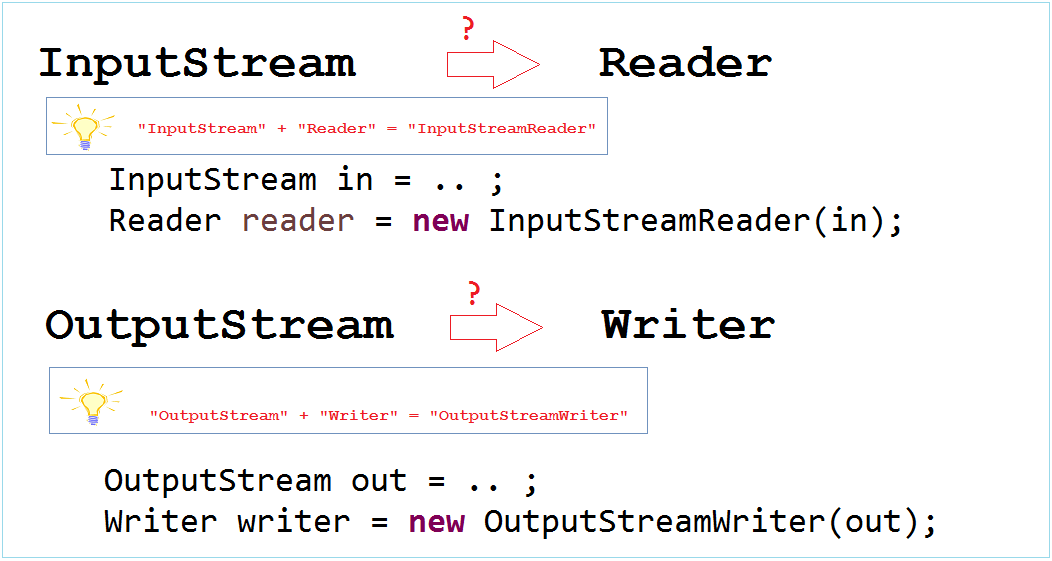In the above examples, we get accustomed with Reader and Writer. The next example allows you actively to read and write in the stream with a clearly specified encoding.
Create file test_utf8.txt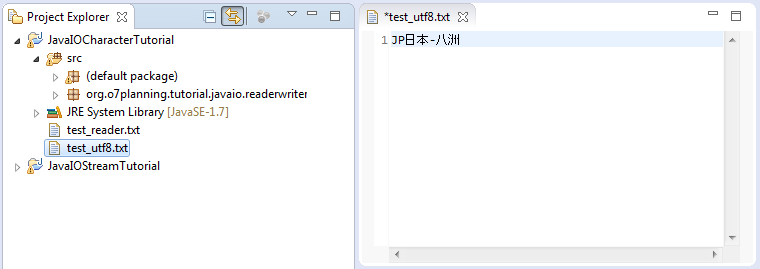test_utf8.txt
``````
JP日本-八洲
``````
When you save, eclipse will ask you what type of encoding you want to save. Select UTF-8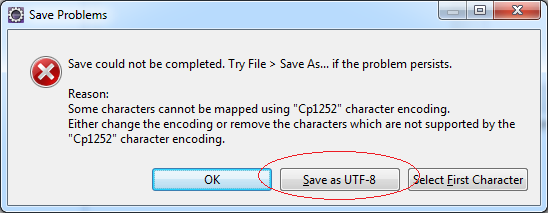``````
package org.o7planning.tutorial.javaio;

import java.io.FileInputStream;
import java.io.IOException;
import java.io.InputStream;

public static void main(String[] args) throws IOException {

// Create binary stream, read a file.
InputStream in = new FileInputStream("test_utf8.txt");

// Create character stream wrap binary stream above.
// Encoding UTF-8

int i = 0;
// cast int to char, and print to the Console.
System.out.println((char) i + " " + i);
}
}
}
``````
Results of running the example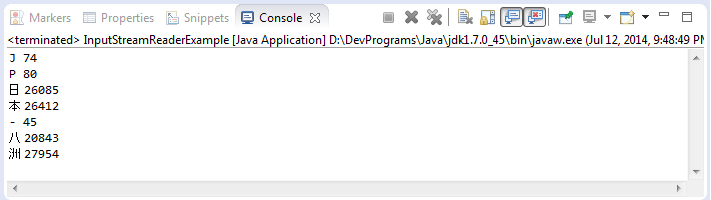The next example, write file encoded with UTF-8.
OutputStreamWriterExample.java
``````
package org.o7planning.tutorial.javaio;

import java.io.File;
import java.io.FileOutputStream;
import java.io.IOException;
import java.io.OutputStream;
import java.io.OutputStreamWriter;
import java.io.Writer;

public class OutputStreamWriterExample {

public static void main(String[] args) throws IOException {

File dir = new File("C:/test");
// Create 'C:test' directory if it does not exists.
dir.mkdirs();
// Create a OutputStream, to write data to a file.
OutputStream out = new FileOutputStream("C:/test/test_write_utf8.txt");

// Create character stream wrap a OutputStream above.
// encoding UTF-8.
Writer writer = new OutputStreamWriter(out, "UTF-8");

String s = "JP日本-八洲";
writer.write(s);
writer.close();
}

}
``````
Results of running the example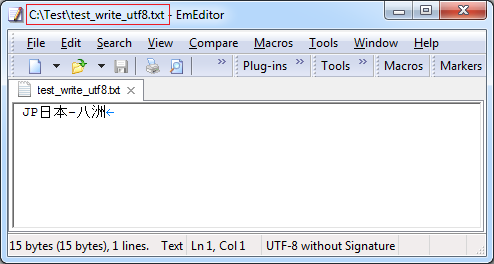If you want to read each line of data of a text file. BufferedReader is a good choice.``````
// Constructor:

// A convenient method of BufferedReader.
// Read a line of text.
``````
Ví dụ:
``````
// Example 1:

// Example 2:
InputStream in = new FileInputStream("C:/test.txt");
``````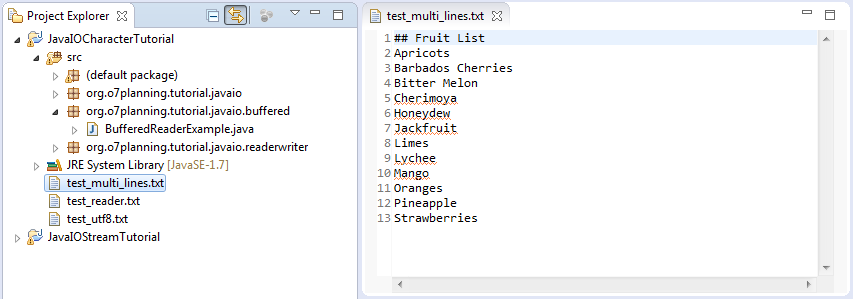test_multi_lines.txt
``````
## Fruit List
Apricots
Bitter Melon
Cherimoya
Honeydew
Jackfruit
Limes
Lychee
Mango
Oranges
Pineapple
Strawberries
``````
``````
package org.o7planning.tutorial.javaio.buffered;

import java.io.FileInputStream;
import java.io.IOException;
import java.io.InputStream;

public static void main(String[] args) throws IOException {

InputStream in = new FileInputStream("test_multi_lines.txt");

String s = null;
int i = 0;

// Read each line of data
// If returns null means ending stream.
while ((s = br.readLine()) != null) {
i++;
System.out.println(i + " : " + s);
}
br.close();
}

}
``````
Results of running the example: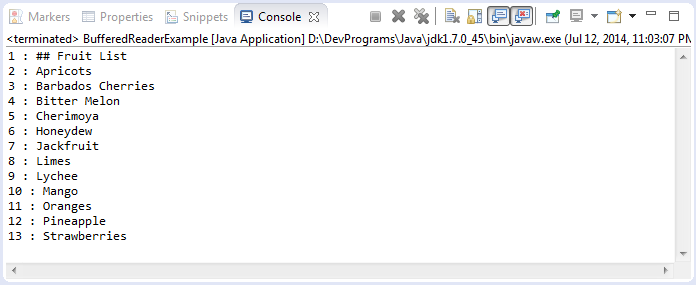## 8- Class java.io.BufferedWriter

BufferedWriter is a direct subclass of the Writer class.
``````
// Create a BufferedWriter object
// by wrapping another Writer object.
public BufferedWriter(Writer out);

// Equivalent to write ('\ n');
public String newLine();
``````
Ví dụ:
``````
// Create Writer object.
Writer w=new FileWriter("C:/test/test_bufferedWriter.txt");

// Create BufferedWriter object wrap a writer.
BufferedWriter bw=new BufferedWriter(w);

bw.write("Hello..");

// Write a new line character.
bw.newLine();
``````

FilterReader is a subclass of Reader. It reads selectively characters on demand. For example, you want to read an HTML document, and ignore the characters in the tags. You need to write a subclass of FilterReader and then use that subclass. You cannot directly use FilterReader since it is a abstract class.Example, create a subclass of FilterReader, to read HTML document but ignore characters in tag.
Input: "<h1>Hello</h1>" ==> output:  "Hello".
``````
package org.o7planning.tutorial.javaio.filter;

import java.io.IOException;

boolean intag = false;

super(in);
}

// Override this method.
// The principle would be:
// Only read characters outside the tags.
@Override
public int read(char[] buf, int from, int len) throws IOException {

int charCount = 0;

while (charCount == 0) {

if (charCount == -1) {
// End the stream.
return -1;
}

int last = from;

for (int i = from; i < from + charCount; i++) {
// If not in an HTML tag.
if (!intag) {
if (buf[i] == '<') {
intag = true;
} else {
buf[last++] = buf[i];
}
} else if (buf[i] == '>') {
intag = false;
}
}

charCount = last - from;
}
return charCount;
}

// Override this method too.
@Override
public int read() throws IOException {
char[] buf = new char;
int result = read(buf, 0, 1);
if (result == -1) {
return -1;
} else {
return (int) buf;
}
}
}
``````
``````
package org.o7planning.tutorial.javaio.filter;

import java.io.IOException;

public static void main(String[] args) throws IOException {

String s = null;
while ((s = br.readLine()) != null) {
System.out.println(s);
}
br.close();
}
}
``````
Results of running the example: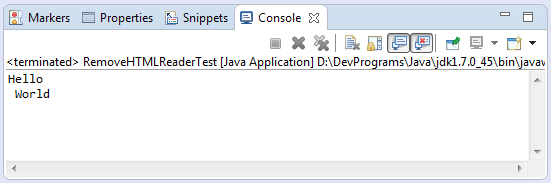## 10- Class java.io.FilterWriter

FilterWriter is a direct subclass of Writer. It writes selectively characters on demand. You need to write a subclass of FilterWriter and override some methods of the FilterWriter class.Example: Characters changed when writing to stream.
Rot13.java
``````
package org.o7planning.tutorial.javaio.filter;

public class Rot13 {

/**
* <pre>
*   a ==> n
*   b ==> o
*   c ==> p
*   d ==> q
*   e ==> r
*   ...
*   y ==> l
*   z ==> m
* </pre>
*/
public static int rotate(int inChar) {
int outChar;

if (inChar >= (int) 'a' && inChar <= (int) 'z') {
outChar = (((inChar - 'a') + 13) % 26) + 'a';
} else if (inChar >= (int) 'A' && inChar <= (int) 'Z') {
outChar = (((inChar - 'A') + 13) % 26) + 'A';
} else {
outChar = inChar;
}
return outChar;
}

// Test
public static void main(String[] args)  {
for(char ch='a'; ch<='z';ch++ ) {
char m= (char)rotate(ch);
System.out.println("ch="+ch+" ==> "+ m);
}

}
}
``````
RotateWriter.java
``````
package org.o7planning.tutorial.javaio.filter;

import java.io.FilterWriter;
import java.io.IOException;
import java.io.Writer;

public class RotateWriter extends FilterWriter {

public RotateWriter(Writer out) {
super(out);
}

// override one or more write methods to perform filtering.
// (override both to be safe)
@Override
public void write(int outChar) throws IOException {
super.write(Rot13.rotate(outChar));
}

@Override
public void write(char[] cbuf, int offset, int length) throws IOException {
char[] tempbuf = new char[length];
for (int i = 0; i < length; i++) {
tempbuf[i] = (char) Rot13.rotate(cbuf[offset + i]);
}
super.write(tempbuf, 0, length);
}

}
``````
RotateWriterTest.java
``````
package org.o7planning.tutorial.javaio.filter;

import java.io.IOException;
import java.io.StringWriter;
import java.io.Writer;

public class RotateWriterTest {

public static void main(String[] args) throws IOException  {
String s="abcdef";

Writer writer= new StringWriter();

RotateWriter rw= new RotateWriter(writer);
rw.write(s.toCharArray(),0,s.length());

rw.close();

String rotateString = writer.toString();
System.out.println("rotateString="+ rotateString);
}
}

``````
Results of running the example: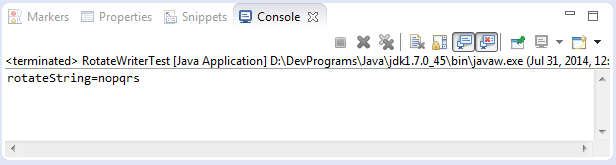The PushbackReader class allows one or more characters to be returned to the stream after reading them. Here are its two constructors:``````

``````
``````
// Pushes back a single character to stream.
public void unread(int c) throws IOException
``````
Example:
``````
package org.o7planning.tutorial.javaio.pushback;

import java.io.IOException;

public static void main(String args[]) throws IOException {
String s = "if (a == 4) a = 0;\\n";
char buf[] = new char[s.length()];
s.getChars(0, s.length(), buf, 0);
int c;
while ((c = f.read()) != -1) {
switch (c) {
// Found character '='
case '=':
// Read next character, (after found '=')
if ((c = f.read()) == '=') {
System.out.print(".eq.");
}

else {
System.out.print("<-");
// Pushes back a single character by copying it to
// the front of the pushback buffer.
// (like - move the cursor back one position).
}
break;
default:
System.out.print((char) c);
break;
}
}
}

}
``````
Results of running the example: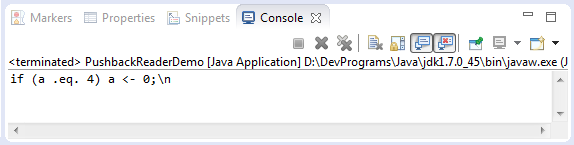## 12- Class java.io.PrintWriter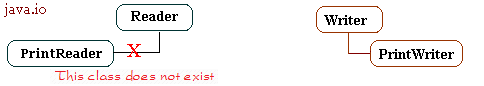``````
// Constructor:
// Wrap a Writer object.
public PrintWriter(Writer out)
public PrintWriter(Writer out,boolean autoFlush)

// Wrap a OutputStream object.
public PrintWriter(OutputStream out)
public PrintWriter(OutputStream out,boolean autoFlush)
public PrintWriter(String fileName)
...

// Method:
public void println(String s)
public void print(char ch)
``````
StackTraceToFile.java
``````
package org.o7planning.tutorial.javaio.printwriter;

import java.io.File;
import java.io.FileWriter;
import java.io.PrintWriter;
import java.io.Writer;

public class StackTraceToFile {

public static void main(String[] args) {
try {
// Do something here.
// Error divided by 0.
int i = 10 / 0;
} catch (Exception e) {
System.out.println("EXCEPTION ....");
try {
File dir = new File("C:/test");
// Create directories if it not exists.
dir.mkdirs();
// Create stream to write data to the file.
Writer w = new FileWriter("C:/test/stackTrace.txt");

// Create PrintWriter object wrap Writer 'w'.
// Data written to the PrintWriter will be pushed into 'w'.
PrintWriter pw = new PrintWriter(w);

// Write 'stack trace' to 'pw'.
e.printStackTrace(pw);
System.out.println("Finish !");
} catch (Exception e1) {
System.out.println("Error:" + e);
}
}
}

}
``````
StackTraceToString.java
``````
package org.o7planning.tutorial.javaio.printwriter;

import java.io.PrintWriter;
import java.io.StringWriter;

public class StackTraceToString {

public static void main(String[] args) {
try {
// Do something here
// Error divided by 0.
int i = 1000 / 0;
} catch (Exception e) {
System.out.println("EXCEPTION ....");
try {
StringWriter sw = new StringWriter();
// Create PrintWriter object wrap StringWriter 'sw'.
// Data written to the PrintWriter will be pushed into 'sw'.
PrintWriter pw = new PrintWriter(sw);

// Write 'stack trace' to 'pw'.
e.printStackTrace(pw);

StringBuffer sb = sw.getBuffer();
String s = sb.toString();
System.out.println("Exception String:");
System.out.println(s);
} catch (Exception e1) {
System.out.println("Error:" + e);
}
}

}

}
``````
Results of running the example: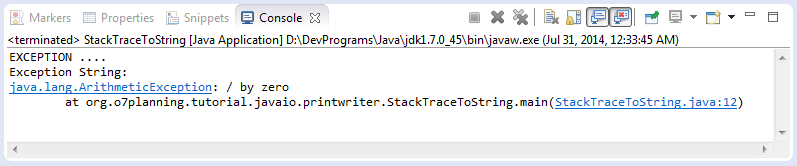``````
package org.o7planning.tutorial.javaio.chararray;

import java.io.IOException;

public static void main(String args[]) throws IOException {

String tmp = "abcdefghijklmnopqrstuvwxyz";
int length = tmp.length();
char c[] = new char[length];
tmp.getChars(0, length, c, 0);

int i;
System.out.println("input1 is:");
while ((i = input1.read()) != -1) {
System.out.print((char) i);
}
System.out.println();
System.out.println("input2 is:");
while ((i = input2.read()) != -1) {
System.out.print((char) i);
}
System.out.println();
}
}
``````
Results of running the example: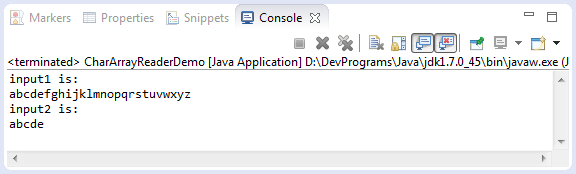## 14- Class java.io.CharArrayWriter``````
// Writes the contents of the buffer to another character stream.
public void writeTo(Writer out) throws IOException
``````
CharArrayWriterDemo.java
``````
package org.o7planning.tutorial.javaio.chararray;

import java.io.CharArrayWriter;
import java.io.File;
import java.io.FileWriter;
import java.io.IOException;

public class CharArrayWriterDemo {

public static void main(String args[]) throws IOException {

char[] c = { 'H', 'e', 'l', 'l', 'o', ' ', 'W', 'o', 'r', 'l', 'd', '!' };
CharArrayWriter out = new CharArrayWriter();

out.write(c);

File dir = new File("C:/test");
dir.mkdirs();

FileWriter f1 = new FileWriter(new File("C:/test/a.txt"));

// Write data of 'out' to 'f1'.
out.writeTo(f1);

FileWriter f2 = new FileWriter(new File("C:/test/b.txt"));

// Write data of 'out' to 'f2'.
out.writeTo(f2);

f1.close();
f2.close();

// Close CharArrayWriter 'out'.
out.close();

FileWriter f3 = new FileWriter(new File("C:/test/c.txt"));

// With CharArrayWriter, after close.
// writeTo(..) method no longer works.
// Also does not cause an exception if you use writeTo (..).
out.writeTo(f3);

System.out.println("Done!");
}
}
``````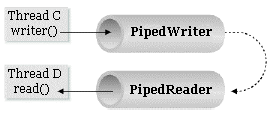• TODO
``````

import java.io.IOException;
import java.io.PipedWriter;

public static void main(String[] args) throws IOException, InterruptedException {
}

private void test() throws IOException, InterruptedException {
// Create a 'pipedWriter',
PipedWriter pipedWriter = new PipedWriter();

// Data writing to 'pipedWriter'
// will automatically appear in 'pipedReader'.

char[] chs = new char[] { 'a', 'a', 'b', 'c' , 'e' };

// Write data to 'pipedWriter'.
for (char ch : chs) {
pipedWriter.write(ch);
}
pipedWriter.close();
}

@Override
public void run() {
try {
int data = 0;
System.out.println((char) data);
}
} catch (Exception e) {
e.printStackTrace();
} finally {
}
}
}

if (is != null) {
try {
is.close();
} catch (IOException e) {
}
}
}

}
``````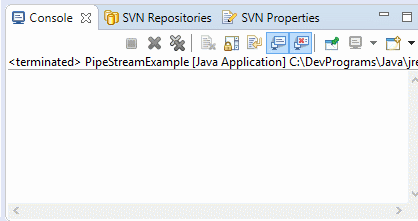``````

import java.io.IOException;
import java.io.PipedWriter;

public static void main(String[] args) throws IOException, InterruptedException {
}

private void test() throws IOException, InterruptedException {
// Create a 'pipedWriter',
PipedWriter pipedWriter = new PipedWriter();

// Data writing to 'pipedWriter'
// will automatically appear in 'pipedReader'.

String[] strs = new String[] { "Hello ", "There", "\n", "I am ", "Tran" };

// Write data to 'pipedWriter'.
for (String str : strs) {
pipedWriter.write(str);
}
pipedWriter.close();
}

@Override
public void run() {
try {
String line = null;
System.out.println(line);
}
} catch (Exception e) {
e.printStackTrace();
} finally {
}
}
}

try {
} catch (IOException e) {
}
}
}

}
``````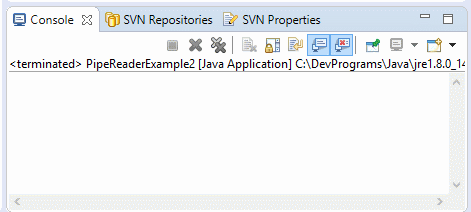## 16- Class java.io.PipedWriter#### View more Tutorials:

Maybe you are interested

These are online courses outside the o7planning website that we introduced, which may include free or discounted courses.